• 利用MATLAB随机函数rand生成comsol随机分布纤维的钢纤维混凝土文件
• 在学习生活中，我们经常性的发现有很多事物背后都有某种规律，而且，这种规律可能符合某种随机分布，比如：正态分布、对数正态分布、beta分布等等。 所以，了解某种分布对一些事物有更加深入的理解并能清楚的阐释...
•matlab
• 离散随机分布

千次阅读 2017-11-06 09:28:42
离散随机分布1.Bernoulli分布：两点分布或者0-1分布。bernoulli试验成功，则Bernoulli随机变量X取值为1，否则X为0。记试验成功概率为θ，即： P(X=1)=θ P(X=0)=1−θ θϵ[0,1] P(X=1)=\theta\ \ \ P(X=0)=1-\...

离散随机分布

1.Bernoulli分布：

两点分布或者0-1分布。bernoulli试验成功，则Bernoulli随机变量X取值为1，否则X为0。记试验成功概率为θ，即：
P(X=1)=θ   P(X=0)=1θ   θϵ[0,1] $P(X=1)=\theta\ \ \ P(X=0)=1-\theta\ \ \ \theta \epsilon[0,1]$
称X服从参数为θ的Bernoulli分布，记为X~Ber(θ)

p(x|θ)={θ   if x = 11θ   if x = 0=θx(1θ)x,  θϵ[0,1] $p(x|\theta) = \left \{ \right.^{\theta\ \ \ if\ x\ =\ 1}_{1-\theta\ \ \ if\ x\ =\ 0} = \theta^x(1-\theta)^x, \ \ \theta \epsilon[0,1]$

Bernoulli均值：
μ=1θ+0(1θ)=θ $\mu = 1*\theta + 0*(1-\theta) = \theta$
Bernoulli方差：
σ2=E(x2)μ2=12θ+02(1θ)θ2=θθ2=θ(1θ) $\sigma^2 = E(x^2) - \mu^2 = 1^2*\theta + 0^2*(1-\theta) - \theta^2 = \theta - \theta^2 = \theta(1-\theta)$

两类分类问题：y|x服从Bernoulli分布，即类别标签y取值为0或1的离散随机变量

2.二项(Binomial)分布：

在抛掷硬币试验中，若只进行一次试验，则为Bernoulli试验。若进行n次试验，则硬币正面向上的数目X满足二项分布，记为: x~ Bin(n,θ)

p(x|n,θ)=Cxnθx(1θ)nx=n!(nx)!x!θx(1θ)nx $p(x|n,\theta) = \mathbb{C}^x_n\theta^x(1-\theta)^{n-x} = \frac{n!}{(n-x)!x!}\theta^x(1-\theta)^{n-x}$
Binomial均值：

已知：
xCxn=nCx1n1 $x\mathbb{C}^x_n = n\mathbb{C}^{x-1}_{n-1}$

μ=0C0nθ0(1θ)n+1C1nθ1(1θ)n1+...+kCknθk(1θ)nk+...+nCnnθn(1θ)0=1C1nθ1(1θ)n1+...+kCknθk(1θ)nk+...+nCnnθn(1θ)0=n(C0n1θ1(1θ)n1+...+Ck1n1θk(1θ)nk+...+Cn1n1θn(1θ)0)=nθ(C0n1θ0(1θ)n1+...+Ck1n1θk1(1θ)nk+...+Cn1n1θn1(1θ)0)=nθ(1θ+θ)n1=nθ $\mu = 0*\mathbb{C}^{0}_{n}\theta^{0}(1-\theta)^{n} + 1*\mathbb{C}^{1}_{n}\theta^{1}(1-\theta)^{n-1} + ... + k*\mathbb{C}^{k}_{n}\theta^{k}(1-\theta)^{n-k} + ... +n*\mathbb{C}^{n}_{n}\theta^{n}(1-\theta)^{0} =1*\mathbb{C}^{1}_{n}\theta^{1}(1-\theta)^{n-1} + ... + k*\mathbb{C}^{k}_{n}\theta^{k}(1-\theta)^{n-k} + ... +n*\mathbb{C}^{n}_{n}\theta^{n}(1-\theta)^{0} = n*(\mathbb{C}^{0}_{n-1}\theta^{1}(1-\theta)^{n-1} + ... + \mathbb{C}^{k-1}_{n-1}\theta^{k}(1-\theta)^{n-k} + ... +\mathbb{C}^{n-1}_{n-1}\theta^{n}(1-\theta)^{0}) = n\theta(\mathbb{C}^{0}_{n-1}\theta^{0}(1-\theta)^{n-1} + ... + \mathbb{C}^{k-1}_{n-1}\theta^{k-1}(1-\theta)^{n-k} + ... + \mathbb{C}^{n-1}_{n-1}\theta^{n-1}(1-\theta)^{0}) = n\theta(1 - \theta + \theta)^{n-1} = n\theta$

Binomial方差：
已知：
x2Cxn=nCx1n1+n(n1)Cx2n2 $x^2\mathbb{C}^x_n = n\mathbb{C}^{x-1}_{n-1} + n(n-1)\mathbb{C}^{x-2}_{n-2}$

σ2=E(x2)μ2 $\sigma^2 = E(x^2) - \mu^2$

E(x2)=ni=0i2Cinpi(1p)ni=C1np(1p)n1+ni=2nCi1n1pi(1p)ni+ni=2(n1)nCi2n2pi(1p)ni=np(1p) $E(x^2) = \sum_{i=0}^n i^2\mathbb{C}_n^ip^i(1-p)^{n-i} = \mathbb{C}_n^1p(1-p)^{n-1} + \sum_{i=2}^n n\mathbb{C}_{n-1}^{i-1}p^i(1-p)^{n-i} + \sum_{i=2}^n(n-1) n\mathbb{C}_{n-2}^{i-2}p^i(1-p)^{n-i} = np(1-p)$

3.多项分布(multinomial)：

假设抛有K个面的的骰子，其中抛掷到第j面的概率为 θj $\theta_j$, 令 θ=(θ1,θ2,...,θk) $\theta=(\theta_1,\theta_2,...,\theta_k)$

若一共抛掷n次， x=(x1,...,xn) $x=(x_1,...,x_n)$为随机变量，其中 xk $x_k$是抛掷到第k面的次数，则x的分布为多项分布，即 x Mu(n,θ) $x~Mu(n,\theta)$

p(x|n,θ)=n!x1!...xkKk=1θxkk $p(x|n,\theta) = \frac{n!}{x_1!...x_k}\prod_{k=1}^K\theta_k^{x_k}$

当n=1时为分类分布，Categorical 分布， x Cat(θ) $x~Cat(\theta)$

展开全文离散随机分布
• 用matplotlib绘制随机分布图 1.创建RandomWalk()类 我们先创建一个随机漫步的类，它可以随机选择前进方向。这个类需要三个属性，其中一个是储存随机漫步次数的变量，其他两个是列表，分别储存随机漫步经过的每个点的...

用matplotlib绘制随机分布图

1.创建RandomWalk()类
我们先创建一个随机漫步的类，它可以随机选择前进方向。这个类需要三个属性，其中一个是储存随机漫步次数的变量，其他两个是列表，分别储存随机漫步经过的每个点的x和y坐标；同时，这个类也包含两个方法，初始化函数和fill_walk()，其中后者计算随机漫步经过的所有点。如下图：

from random import choice

class RandomWalk():
"""一个生成随机漫步数据的类"""

def __init__(self,num_points=5000):
"""初始化随机漫步的属性"""
self.num_points = num_points

#所有随机漫步都始于(0,0)
self.x_values = 
self.y_values = 

def fill_walk(self):

"""计算随机漫步包含的所有点"""

#不断漫步，直到列表达到指定长度
while len(self.x_values) < self.num_points:

#决定前进方向以及沿这个方向前进的距离
x_direction = choice([1,-1])
x_distance = choice([0,1,2,3,4])
x_step = x_direction * x_distance

y_direction = choice([1,-1])
y_distance = choice([0,1,2,3,4])
y_step = y_direction * y_distance

#拒绝原地踏步
if x_step == 0 and y_step == 0:

continue

#计算下一个点的x和y值
next_x = self.x_values[-1] + x_step
next_y = self.y_values[-1] + y_step

self.x_values.append(next_x)
self.y_values.append(next_y)

在这里choise()函数是随机选择的一个方法。然后有兴趣可以研究一下随机漫步的算法，我就不解释了。

2.绘制随机分布图
下面我们绘制一个随机分布图，并设置一个循环，让你可以看到不同的分布图。

import matplotlib.pyplot as plt

from random_walk import RandomWalk

#只要程序处于活动状态，就不断地模拟随机漫步
while True:

#创建一个RandomWalk实例，并将其包含的点都绘制出来
rw = RandomWalk()
rw.fill_walk()
plt.scatter(rw.x_values,rw.y_values,s=15)
plt.show()

keep_running = input("Make another walk? (y/n):")
if keep_running == 'n':
break

结果：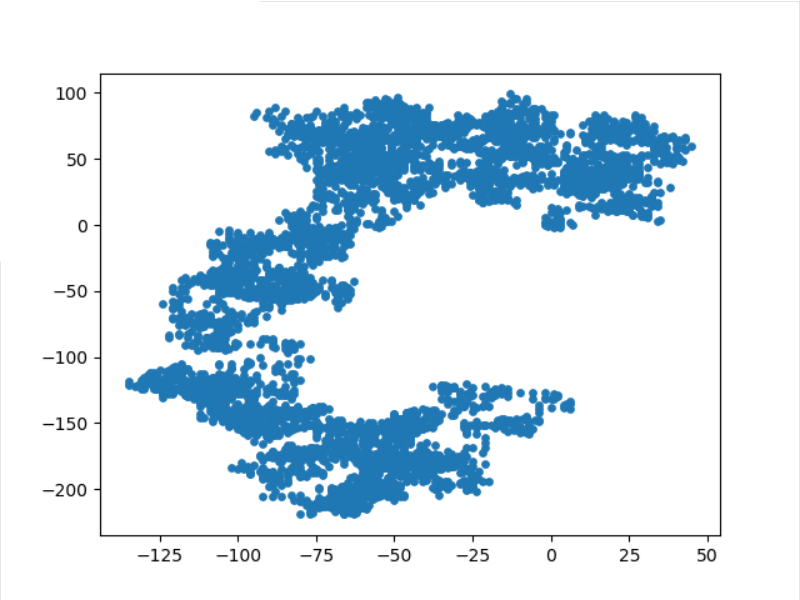然后就绘制出来了。
（参考资料《Python编程从入门到实践》）

展开全文• Java中实现正态随机分布

万次阅读 2018-08-24 17:00:52
这次内容分为两部分，如果你对算法怎么实现没兴趣的话，看看简单版如何调用库函数就好了，如果你和我一样对算法又有那么点兴趣，那就...//标准正态随机分布 public static double StandardNormalDistribution(){ ...

这次内容分为两部分，如果你对算法怎么实现没兴趣的话，看看简单版如何调用库函数就好了，如果你和我一样对算法又有那么点兴趣，那就接着看看进阶版，看不懂也不亏嘛。

简单版

如果不想自己写的话，Java util库中早就有提供好的标准正态分布的随机函数：

//标准正态随机分布
public static double StandardNormalDistribution(){
java.util.Random random = new java.util.Random();
return random.nextGaussian();
}

库里只有标准正态分布N(0,1)，那么想得到满足N(u,v)的随机数怎么办呢，利用N(0,1)有个近似的简单算法：

//普通正态随机分布
//参数 u 均值
//参数 v 方差
public static double NormalDistribution(float u,float v){
java.util.Random random = new java.util.Random();
return Math.sqrt(v)*random.nextGaussian()+u;
}

有人可能会怀疑上面   Math.sqrt(v)*random.nextGaussian()+u  这个公式到底靠谱吗？我们先来证明下吧：

同时，我们在Matlab里面验证一下，附上Matlab代码：

%%%%随机数数量
L=1000000;
x=zeros(1,L);
y=zeros(1,L);

%%%%均值
u=10;
%%%%方差
v=10;

%%%%normrnd是正态分布函数
for i=1:length(x)
x(i)=u+sqrt(v)*normrnd(0,1);
y(i)=normrnd(u,v);
end

figure(1);
hist(x,1000);
title('近似算法');

figure(2);
hist(y,1000);
title('标准算法');

来看结果：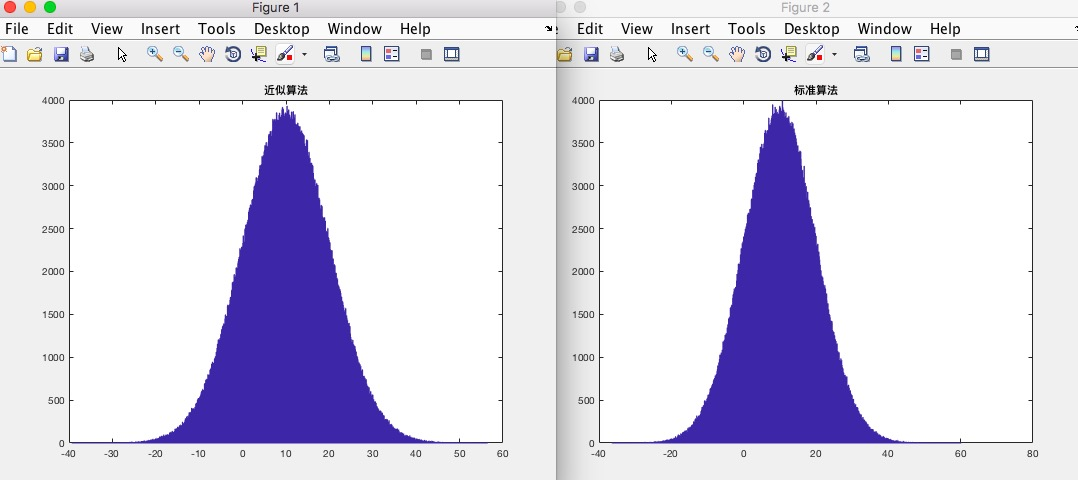可见图像还是非常接近的，说明近似效果还是相当不错的。

进阶版

如果你跟我一样很闲，想要自己实现下正态分布的算法，那就接着看吧。我这里主要介绍Box–Muller算法和中心极限定理。

Box–Muller算法

Box–Muller算法是由 George E. P. Box 与 Mervin E. Muller 在1958年提出的，证明过程很复杂这里就不提了，但是公式却很简单，看公式：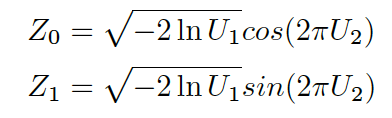感谢网友提供的图。这个公式主要利用了两个均匀分布产生的随机数U1，U2。Z0和Z1实现的效果是一致的，我们在代码中实现一下：

//生成两个(0~1)的随机数 x1,x2;
double x1=Math.Random();
double x2=Math.Random();

double Z0=Math.sqrt(-2*Math.log(x1))*Math.cos(2*Math.PI*x2);
double Z1=Math.sqrt(-2*Math.log(x1))*Math.sin(2*Math.PI*x2);

还是非常简单的。那么真实的效果又如何呢，在Matlab里面验证一下：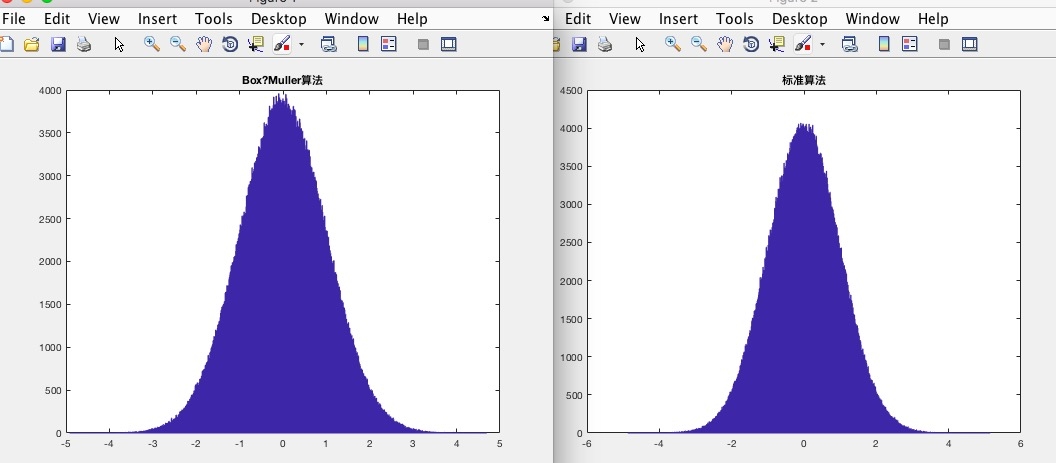用了一百万个随机数据画出的分布直方图，可见还是非常不错的。当然这里实现的还是标准正态分布N(0,1)，若要得到一般的正态分布N(u,v)，还需要利用之前的公式再转换一下。

中心极限定理

中心极限定律很有意思，简而言之呢就是说从各种独立分布中都可以找到一个规律，这个规律近似满足正态分布，具体的推导方面可以自行研究。

假设有一个独立随机的分布 X={x1,x2,x3,...,xn}

可知X的期望 E{X}=u

X的方差 D{X}=v

n越大，Y的分布就越近似于正态分布。而当n接近无穷大时，得到的Y可以看作标准正态分布N(0,1)。

那么我们就试着用均匀分布来实现正态分布：

public static float getStandardGaussian(){
//(0~1)均匀分布均值为0.5
float u=0.5;
//(0~1)均匀分布方差为1/12
float v=1/12;

//n取100个点
int n=100;

float sum=0;
for(int i=0;i<n;i++){
sum+=Math.Random();
}

float y=(sum-n*u)/Math.sqrt(n*v);
return y;
}

来吧，看看Matlab的验证效果如何：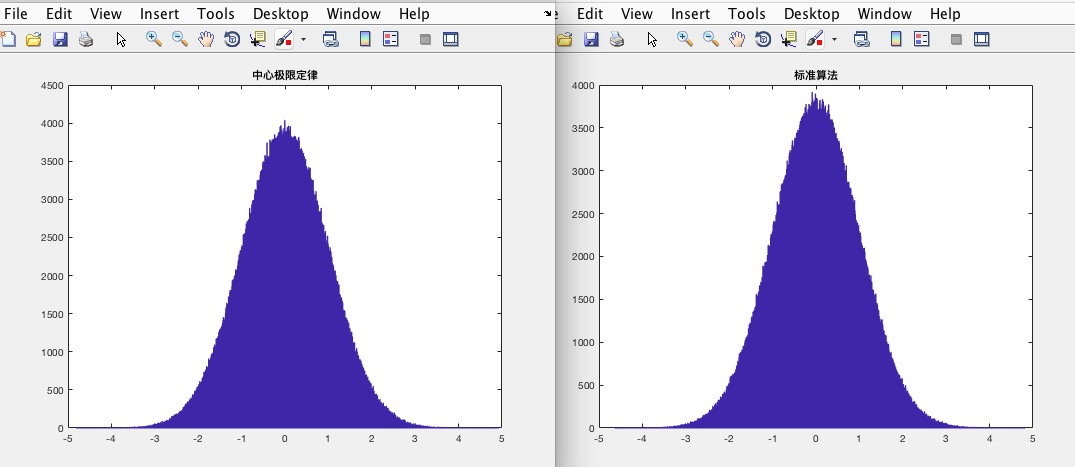还是很不错的。不过有个缺点就是每次计算n 遍，没有Box–Muller算法计算的快。同样的，计算得到的是标准正态随机分布，一般的分布还是需要转换一下才行。

展开全文Java 正态分布 算法
• 1.产生[0 N-1]区间的一个随机整数 ...4.产生服从某个分布的概率随机数 1.产生[0 N-1]区间的一个随机整数 #include <iostream> #include<stdlib.h> using namespace std; int main() { srand((int)ti...

1.产生[0 N-1]区间的一个随机整数
2.产生一个[0 1]区间的随机小数
3.产生一个[0 N-1]区间的随机序列（常用于RANSAC）
4.产生服从某个分布的概率随机数

1.产生[0 N-1]区间的一个随机整数

#include <iostream>
#include<stdlib.h>
using namespace std;
int main() {
srand((int)time(0)); //如果不加这个，重新运行程序得到的是一样顺序的随机数
int N=51;
for (int i = 0; i < 10; i++) {
cout << random()%(N-1) <<endl;
}
}

2.产生一个[0 1]区间的随机小数

#include <iostream>
#include <vector>
#include<stdlib.h>
using namespace std;
int main() {
srand((int)time(0));
vector<int> nums(10,0);
for (int i = 0; i < 100000; i++) {
//(double)random()/(RAND_MAX)就是产生的随机小数
int k=((double)random()/(RAND_MAX)) /0.1;
nums[k]++;
}
for(int i=0;i<nums.size();i++)
{
cout<<"nums["<<i<<"] = "<<nums[i]<<endl;
}
}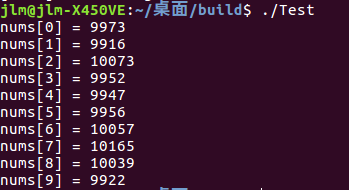3.产生一个[0 N-1]区间的随机序列
意思就是在[0 N-1]之间的N个整数中随机挑取n个不重复的整数，在RANSAC中经常被使用到，ORBSLAM2中的RANSAC八点法求F矩阵使用到了这种方法。
下面代码展示了从0-99中随机挑选8个不重复的数的组合

#include <iostream>
#include<vector>
#include<stdlib.h>
using namespace std;
int main() {
srand((int)time(0));
int N=100;
int n=8;
vector<int> vAllIndices(N);
vector<int> vAvailableIndices(n);
cout<<"vAllIndices = "<<vAllIndices<<endl;
for(int i=0;i<vAllIndices.size();i++)
{
vAllIndices[i]=i;
}
for(int i=0;i<vAvailableIndices.size();i++)
{
int idx=random()%vAllIndices.size();
vAvailableIndices[i]=vAllIndices[idx];
vAllIndices[idx]=vAllIndices.back();
vAllIndices.pop_back();
}
for(int i=0;i<vAvailableIndices.size();i++)
{
cout<<vAvailableIndices[i]<<" ";
}
cout<<endl;
}

这段代码选择了多组这样的组合，适用于多次的RANSAC

#include <iostream>
#include<vector>
#include<stdlib.h>
using namespace std;
int main() {
srand((int)time(0));

int N=100;  //从0-N-1中产生随机数
int n=8;    //一次RANSAC需要的个数
int iteration=300;//准备进行iteration次RANSAC

vector<int> vOriginalIndices(N);
vector<int> vProcessingIndices(N);
vector<vector<size_t>> vResultIndices(iteration,vector<size_t>(n,0));

for(int i=0;i<vOriginalIndices.size();i++)
{
vOriginalIndices[i]=i;
}

for(int i=0;i<iteration;i++)
{
vProcessingIndices=vOriginalIndices;
for(int j=0;j<n;j++)
{
int idx=random()%vProcessingIndices.size();
vResultIndices[i][j]=vProcessingIndices[idx];
vProcessingIndices[idx]=vProcessingIndices.back();
vProcessingIndices.pop_back();
}
}
}

4.产生服从某个分布的概率随机数
读别人代码时发现他们经常使用随机数发生器来产生数据，简单地总结下用法
包含的头文件是#include<random>
应用上就是定义一个generator，这种随机数发生器的种子应该就够用了
std::default_random_engine generator;
然后定义一个分布函数xy_rand，此处定义了一个均匀分布，需要定义的参数是均匀分布的上下限 std::uniform_real_distribution<double> distribution_uniform(-4, 4.0);
另一种经常使用的分布是高斯分布，需要定义的参数是均值和标准差（不是方差），在用作噪声时均值通常被设置为0，
std::normal_distribution<double> distribution_normal(0., 10);

示例函数，在x轴方向上产生均匀分布，y方向标准正态分布，然后描绘二位坐标点的分布情况

#include<iostream>
#include<fstream>
#include<random>

using namespace std;

int main()
{
ofstream out("point_random.txt");
std::default_random_engine generator;
std::uniform_real_distribution<double> distribution_uniform(-4, 4.0);
std::normal_distribution<double> distribution_normal(0., 1);
cout<<"distribution.stddev() = "<<distribution_normal.stddev()<<endl;
for(size_t i=0;i<10000;i++)
{
out<<distribution_uniform(generator)<<" "<<distribution_normal(generator)<<endl;
}
}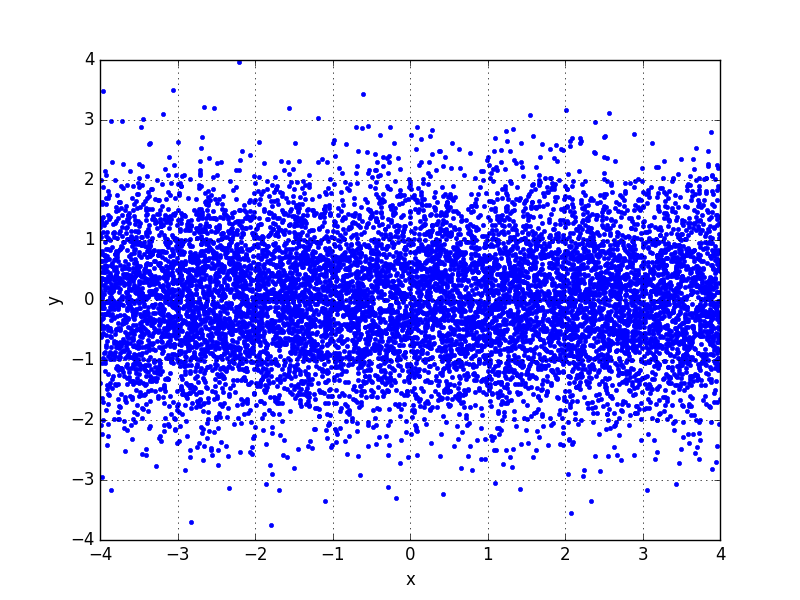然后把y轴上的正态分布的标准差改为10，产生了下面这种情况，符合标准差越大，数据越分散的高斯性质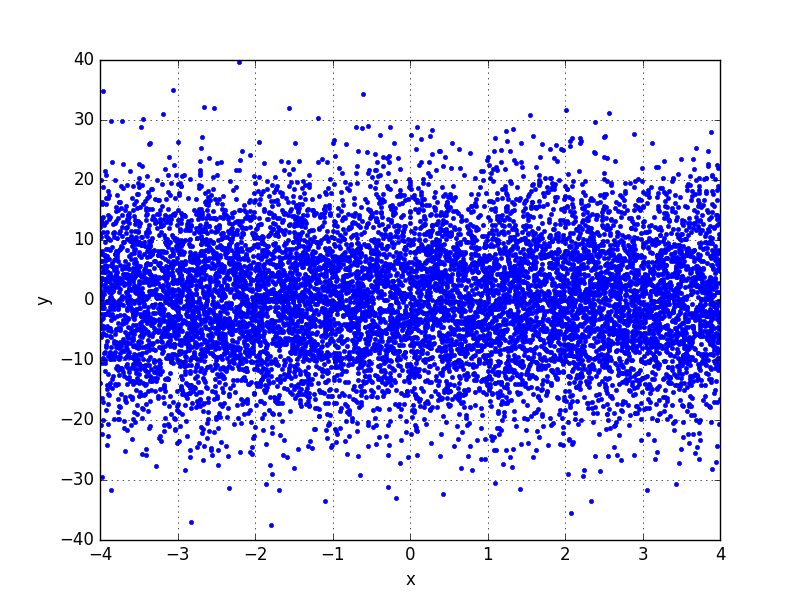展开全文• 用python生成各种随机分布图

万次阅读 多人点赞 2017-06-06 23:37:08
在学习生活中，我们经常性的发现有很多事物背后都有某种规律，而且，这种规律可能符合某种随机分布，比如：正态分布、对数正态分布、beta分布等等。 所以，了解某种分布对一些事物有更加深入的理解并能清楚的阐释...python 帕累托分布 正态分布 gamma分布 beta分布
• 前面的文章中主要就线性回归进行了模拟，这篇文章重点讨论一下学习和训练过程中训练数据的问题。并对随机分布的噪声如何生成以及结果展示进行确认。
• R语言中实现随机分布

千次阅读 2018-09-19 16:07:55
在R中各种概率函数都有统一的形式，即一套统一的 前缀+分布函数名： 如： 1. r*** function (for "random"随机函数), a d*** function (for "density"密度函数), a p*** (for "...R random
• 如果y在a,b之间随机均匀分布，则y的方差为（a-b)**2/12,y的期望为（a+b)／2
• 原文地址：利用R生成随机分布的方法">[整理] 利用R生成随机分布的方法作者：周庭锐教授[整理] 利用R生成随机分布的方法 文/周庭锐 夜里复习各种统计分布的模拟、拟合、验证的R编程，顺手整理一下。 （不懂怎么一回...
•  在半径为1的圆中随机选取一点 问题分析  假设圆心所在位置为坐标元点(0, 0)。 方法1  在x轴[-1, 1]，y轴[-1, 1]的正方形内随机选取一点。  然后判断此点是否在圆内（通过计算此点到圆心的距离）。 ...
• 本代码主要利用MATLAB工具实现MATLAB——创建标准正态分布随机矩阵，简单明了，易于理解
• 方案一： import numpy as np array = np.arange(0, 10, 1) print("原来数据顺序:") print(array) state = np.random.get_state() np.random.shuffle(array) ...print("打乱原顺序，新的随机数据:") print(ar...Python
• 对概率密度函数积分归一化得到概率函数，然后采用概率函数反函数生成随机分布； 代码实现 void GenerateDirection() { TRandom3 rndm; TH1D *h1 = new TH1D("","",400,0.,3.1415/2); for(int i=0;i;
• 题：设随机变量 独立同分布且具有相同的分布函数，证明：   证明： 在以下证明中假设f(x), F(x) 分别为 共同的概率密度和分布函数 步骤一： 大于 到 中的全部值也就是说对于任意一个 均小于 ，所以原式...
• 程序实现泊松随机分布

千次阅读 2015-01-26 09:58:44
泊松分布适合于描述单位时间（或空间）内随机事件发生的次数。如某一服务设施在一定时间内到达的人数，电话交换机接到呼叫的次数，汽车站台的候客人数，机器出现的故障数，自然灾害发生的次数，一块产品上的缺陷数，...
• 基于COMSOL5.5版本，利用java script构建apps创建随机几何，基于该模型，可学习COMSOL App的使用以及java script的联用，对于模型的描述在官网上可找到相关博客。
• 用蒙特卡洛法验证2d平面上随机实数的分布是否为随机分布，用VB编程实现怎么做？
• 利用Metrepolis Rejection方法生成符合任意概率分布的伪随机伪随机数生成
• 对离散型随机分布的理解

千次阅读 2012-05-05 23:02:30
01分布是比较简单的离散型分布，是伯努利分布的一个特例。伯努利分布：F(x)= ∑(k:0->x){C(n,k) p^k(1-p)^(n-k)},取n=1,即做一次伯努利试验，则得01分布。当n->∞时，p比较小的时候，P(X=k)=C(n,k) p^k(1-p)^(n-k,设...数据挖掘 c
• 10个随机分布的坐标点 Demo #!/usr/bin/python3 from random import uniform ''' uniform(a,b)生成[a,b)内的一个随机数 ''' r=[(uniform(0,10),uniform(0,10)) for _ in range(10)] print(r) 运行结果 [(3....python
• 离散型随机变量的概率分布

千次阅读 2020-12-16 16:51:59
这一小节我们一起学习几个离散型随机变量里的特殊的概率分布：几何分布、二项分布和泊松分布。 几何分布 乘风破浪的姐姐最近正在热播，还记得万茜小姐姐第一次个人solo的那段吉他弹唱吗？第一次她在台上弹错了，第二...离散型随机变量 概率分布 几何分布 二项分布 泊松分布...